Note: 本文基于 3GPP Rel15 (2020-09) 版本规范。

SRS 用于上行信道信息获取、满足信道互易性时的下行信道信息获取以及上行波束管理。

NR 定义了 3 种类型的 SRS 传输：周期性 SRS，半持续性 SRS 和非周期性 SRS，通过为 SRS 资源集和 SRS 资源配置关于时域类型的高层参数 resourceType 来实现。

SRS 资源集配置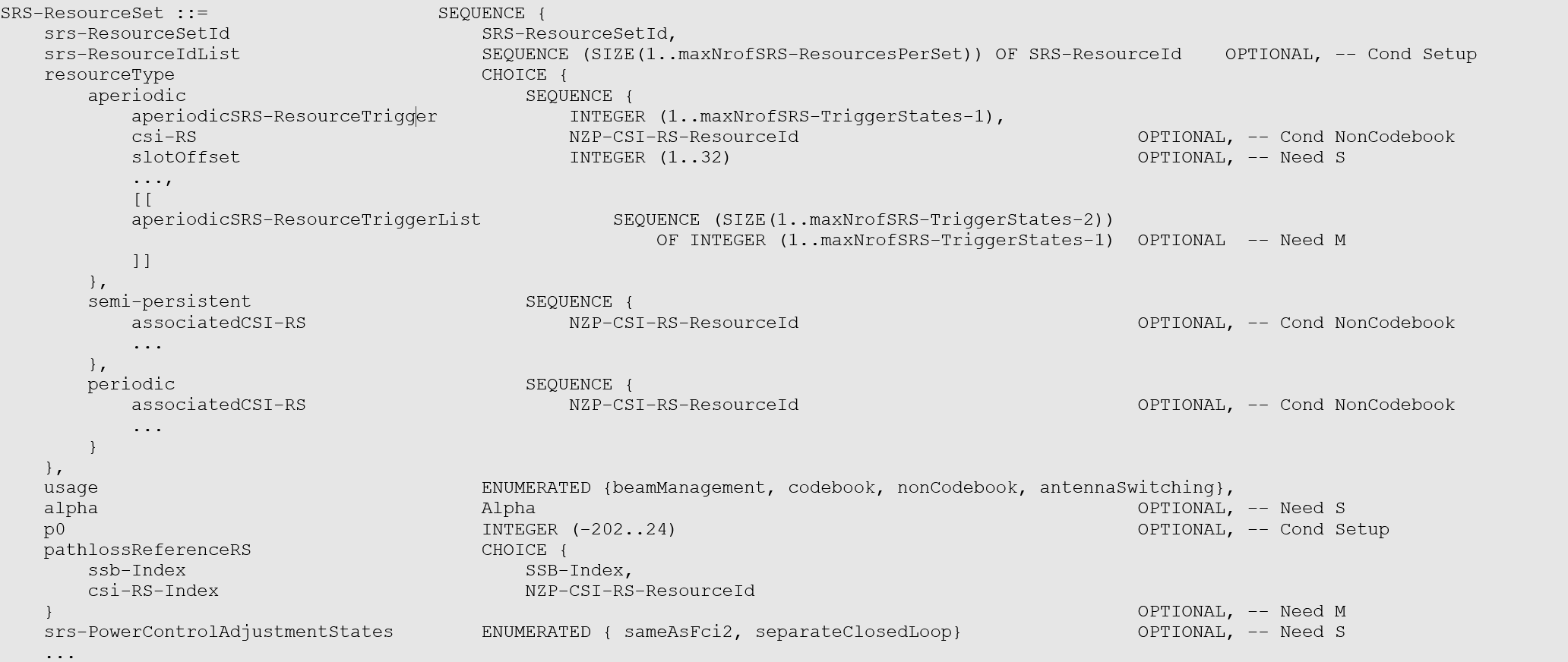SRS 资源配置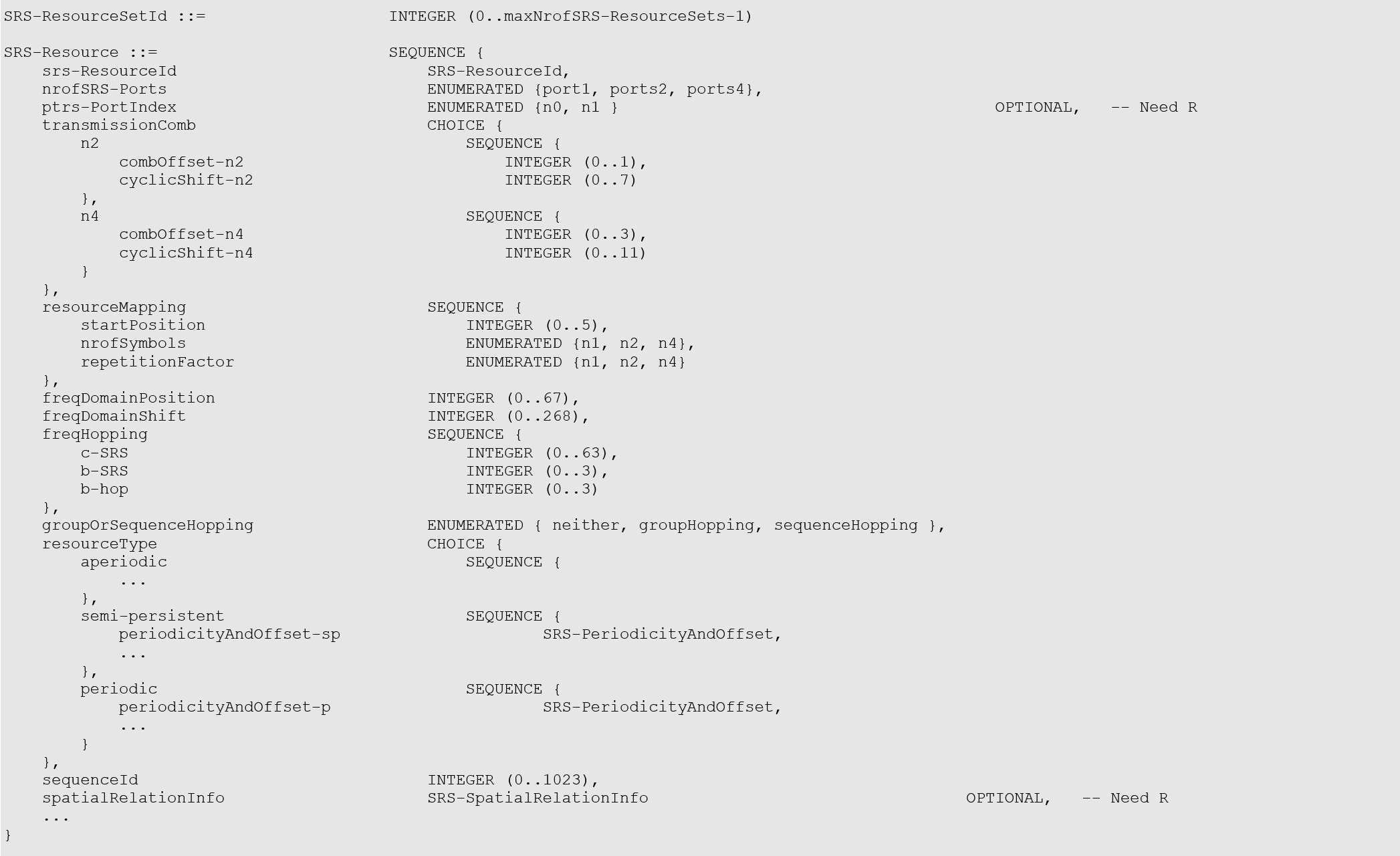• 周期性 SRS。时域类型被配置为周期 SRS 资源的所有参数由高层信令配置，UE根据所配置的参数进行周期性发送。同一个 SRS 资源集内的所有 SRS 资源具有相同的周期性。考虑到 NR 系统支持各种子载波间隔，不同子载波间隔对应的时隙时长不同，周期 SRS 资源的周期以及周期内的偏移以时隙为单位进行配置。周期 SRS 资源可配置的最小周期为 1 个时隙，最大周期为 2560 个时隙。
• 半持续性 SRS。时域类型被配置为半持续 SRS 资源在激活期间也是周期性发送。它与周期性 SRS 的区别在于 UE 在接收到关于半持续 SRS 资源的高层信令配置后不发送 SRS，只有在接收到 MAC 层发送的关于半持续 SRS 资源的激活信令后才开始周期性地发送半持续 SRS 资源对应的 SRS ，在收到 MAC 层发送的半持续 SRS 资源的去激活命令后停止发送 SRS。因此，相对于周期性 SRS 资源，半持续 SRS 资源的配置以及激活、去激活相比高层信令（RRC信令）更快，更灵活，适用于要求时延较低的业务的快速传输。与周期性 SRS 资源类似，基站通过高层信令为半持续 SRS 资源配置周期和周期内的偏移，同一个 SRS 资源集内的所有SRS资源具有相同的周期性。
• 非周期性 SRS。时域类型被配置为非周期 SRS 资源通过 DCI 信令激活。UE 每接收到一次触发非周期 SRS 资源的 SRS 触发信令，UE 进行一次所触发的 SRS 资源对应的 SRS 发送。DCI 中的 SRS 触发信令包含 2 个比特（如表 Table 7.3.1.1.2-24 所示），2 个比特可表示的 4 个状态。其中中的 1 个状态表示不触发非周期 SRS 发送，其他 3 个状态分别表示触发第一、第二、第三个 SRS 资源组；一个状态可以触发一个或多个 SRS 资源集，一个状态对应的多个 SRS 资源集可以对应多个载波。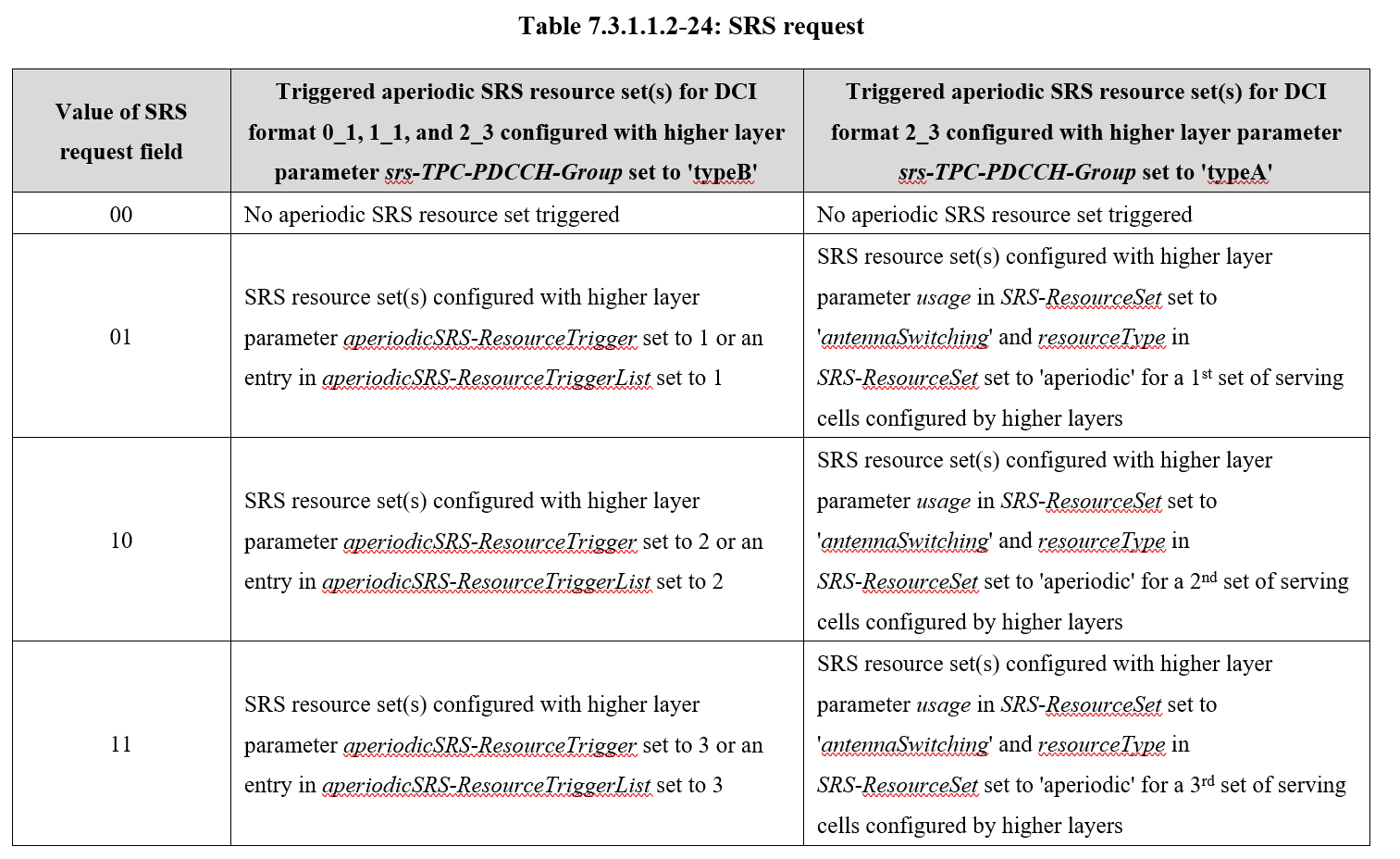# SRS 资源定义

SRS 资源由「天线端口数目 $$N_{\mathrm{ap}}^{\mathrm{SRS}}$$，连续的 OFDM 符号数目 $$N_{symb}^{SRS}$$，时域起始符号 $$\ l_0$$ 和频域起始位置 $$k_0$$」四个信息共同确定。

• 如果高层参数 SRS-ResourceSet 中的 usage 未配置为 “nonCodebook”，则 $$N_{\mathrm{ap}}^{\mathrm{SRS}}$$ 由 nrofSRS-Ports 确定。

• 如果高层参数 SRS-ResourceSet 中的 usage 配置为 “nonCodebook”，则 $$N_{\mathrm{ap}}^{\mathrm{SRS}}$$ 取决于 section 5.2.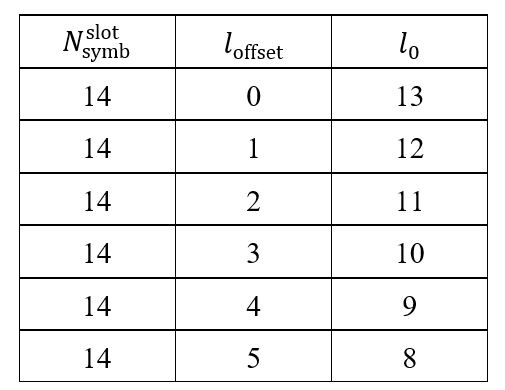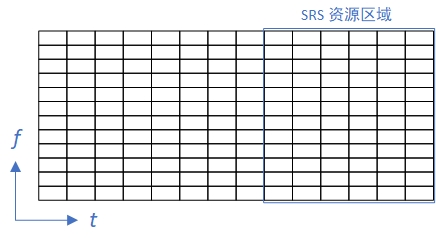# SRS 序列生成

$r^{\left(p_i\right)}\left(n,l'\right)=r_{u,v}^{\left(\alpha_i,\delta\right)}\left(n\right)$

$0\le n\le M_{\mathrm{sc},b}^{\mathrm{SRS}}-1$

$l'\in\left\{0,1,\ldots,N_{\mathrm{symb}}^{\mathrm{SRS}}-1\right\}$

$$r_{u,v}^{\left(\alpha_i,\delta\right)}\left(n\right)$$ 是 扩展 ZC 序列，序列长度为 $$M_{\mathrm{sc},b}^{\mathrm{SRS}}$$，其中 $$\delta={\mathrm{log}}_\mathrm{2}\left(K_{\mathrm{TC}}\right)$$$$\alpha_i$$ 是对应于天线端口 $$p_i$$ 的循环移位。$$l'$$ 是 SRS 资源的 OFDM 符号索引。传输梳齿数目 $$K_{\mathrm{TC}}$$ 由高层参数 transmissionComb 确定，循环移位 $$\alpha_i$$ 由下式给出：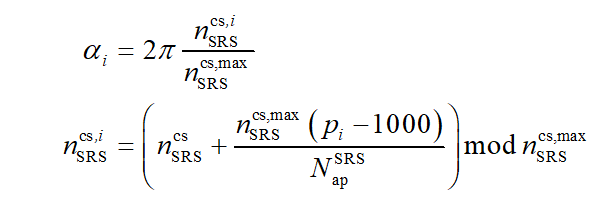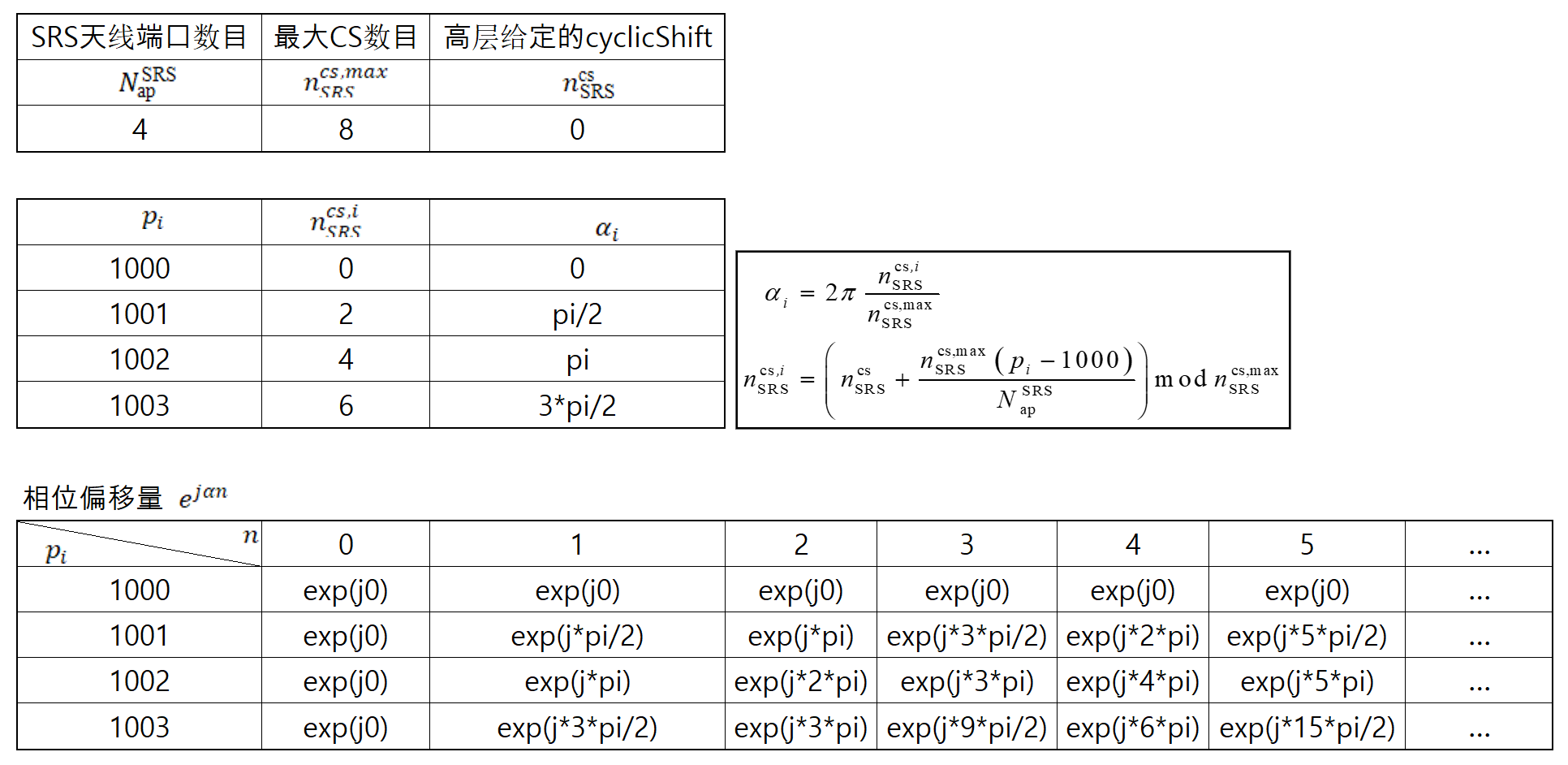SRS 的基序列被分成若干组，每组包含若干序列。如果基站给终端配置为 groupOrSequenceHopping = neither，则每次终端发送的 SRS 序列不变；如果基站给终端配置为 groupOrSequenceHopping = groupHopping or sequenceHopping，则每次终端发送上行 SRS 时按照以下规则采用不同的序列。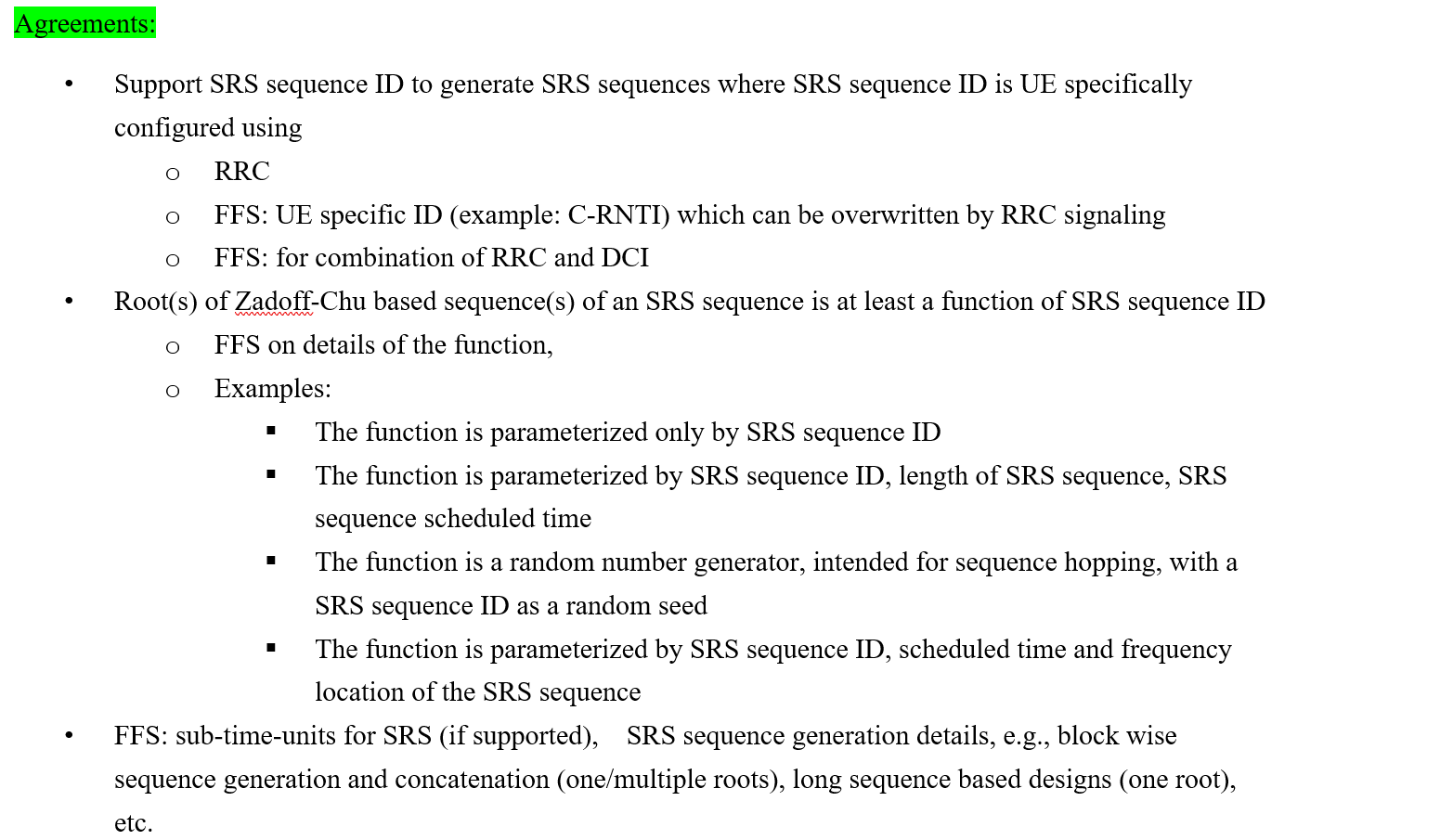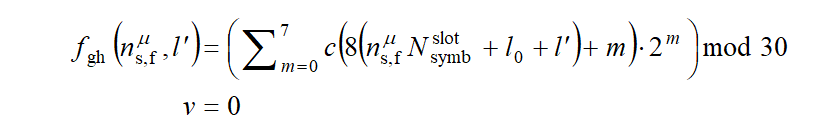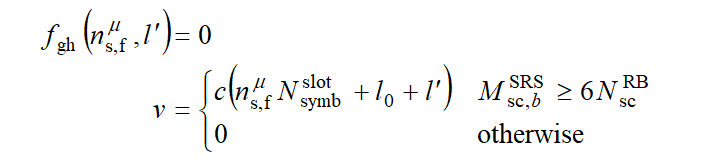# 映射到物理资源

## SRS 频域资源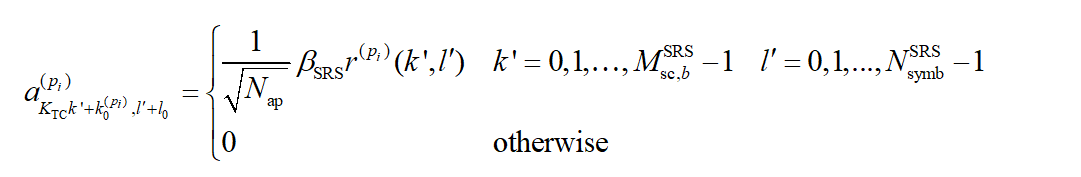SRS 序列长度等于 SRS 资源在一个符号内占用的子载波数目，具体定义为

$M_{\mathrm{sc},b}^{\mathrm{SRS}}=\frac{m_{\mathrm{SRS},b}N_{\mathrm{sc}}^{\mathrm{RB}}}{K_{\mathrm{TC}}}$

$$m_{\mathrm{SRS},b}$$ 通过查 Table 6.4.1.4.3-1 得到，其中 $$b=B_{\mathrm{SRS}}$$$$B_{\mathrm{SRS}}\in\left\{0,1,2,3\right\}$$ 由高层参数 freqHopping 中的字段 b-SRS 给定，$$C_{\mathrm{SRS}}\in\left\{0,1,...,63\right\}$$ 由高层参数 freqHopping 中的 c-SRS 给定。查表时通过 $$C_{\mathrm{SRS}}$$ 选中某一行，再通过 $$B_{\mathrm{SRS}}$$ 选中某一列，即可最终确定 SRS 的带宽信息。 根据 Table 6.4.1.4.3-1，NR 系统支持 64 种 SRS 带宽配置方式，一个 SRS 资源可配置的最小带宽为 4 个 RB，最大带宽为 272 个RB。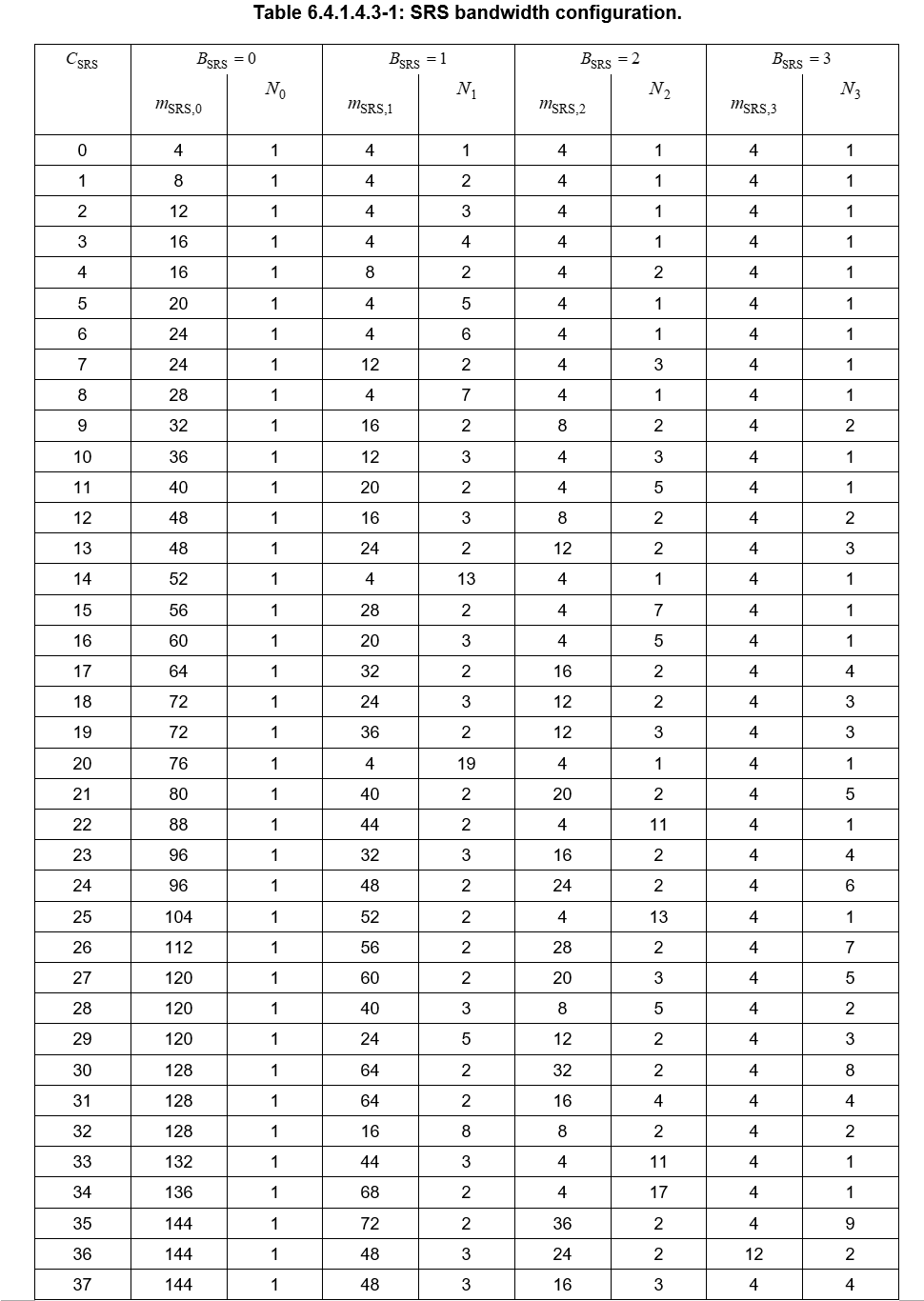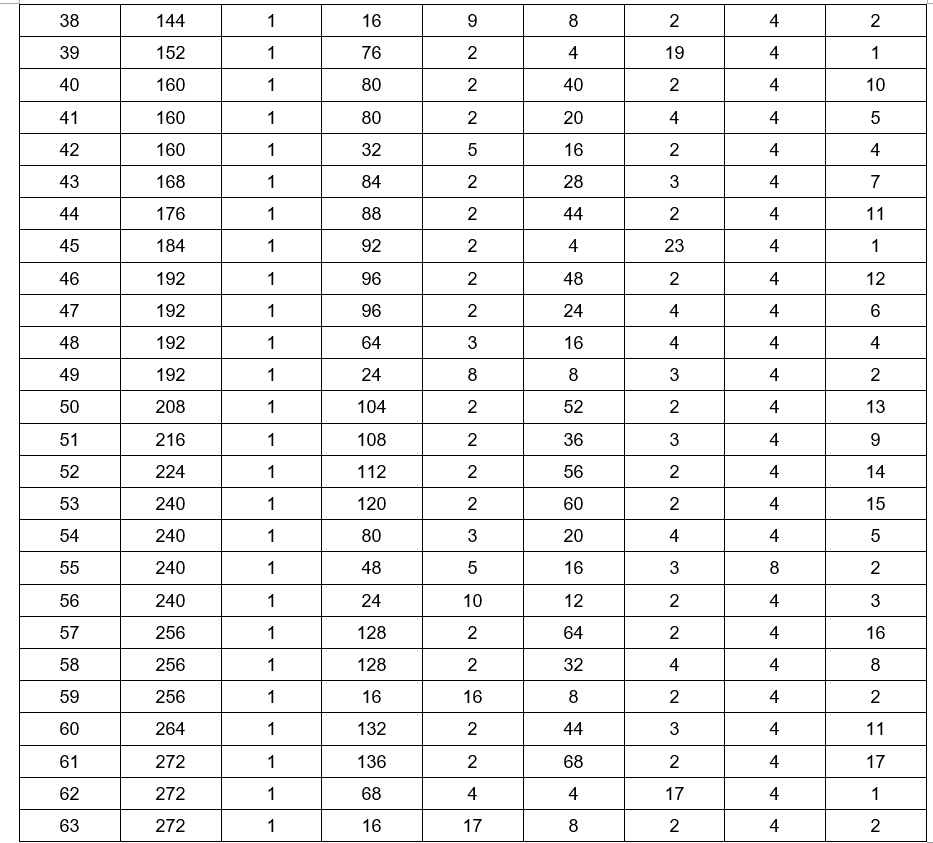$k_0^{(p_i)} = {\bar{k}}_0^{\left(p_i\right)} + \sum_{b=0}^{B_{\mathrm{SRS}}}{K_{\mathrm{TC}}M_{\mathrm{sc},b}^{\mathrm{SRS}}}n_b$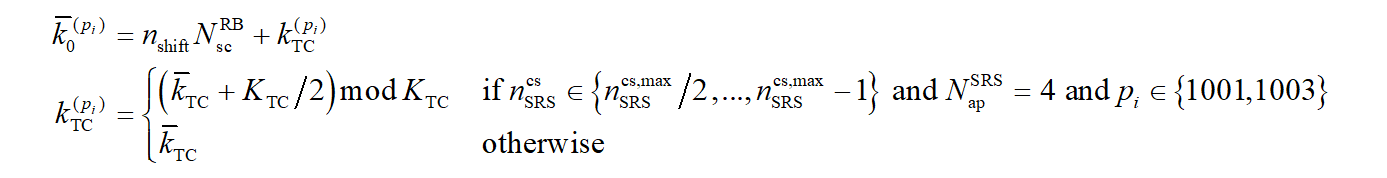SRS 是否跳频由高层参数 freqHopping 中的字段 b-hop 决定。$$b_{\mathrm{hop}}$$ 取值范围 0~3。

$n_b=\left\lfloor\frac{4n_{\mathrm{RRC}}}{m_{\mathrm{SRS,}b}}\right\rfloor\ mod{\ N}_b$

$n_b=\left\{\begin{matrix}\left\lfloor\frac{4n_{\mathrm{RRC}}}{m_{\mathrm{SRS},b}}\right\rfloor\mathrm{\ mod\ }\ N_\mathrm{b}&b\le b_{\mathrm{hop}}\\\left(F_b\left(n_{\mathrm{SRS}}\right)+\left\lfloor\frac{4n_{\mathrm{RRC}}}{m_{\mathrm{SRS},b}}\right\rfloor\right)\mathrm{\ mod\ }\ N_\mathrm{b}&\mathrm{otherwise}\\\end{matrix}\right.$

$F_b(n_{\mathrm{SRS}})=\left\{\begin{matrix}(N_b/2)\left\lfloor\frac{n_{\mathrm{SRS}}mod{\Pi_{b'=b_{\mathrm{hop}}}^b}N_{b'}}{\Pi_{b'=b_{\mathrm{hop}}}^{b-1}N_{b'}}\right\rfloor+\left\lfloor\frac{n_{\mathrm{SRS}}mod{\Pi_{b'=b_{\mathrm{hop}}}^b}N_{b'}}{2\Pi_{b'=b_{\mathrm{hop}}}^{b-1}N_{b'}}\right\rfloor&\mathrm{if\ }N_b\mathrm{\ even\ }\ \\\left\lfloor N_b/2\right\rfloor\left\lfloor n_{\mathrm{SRS}}/\Pi_{b'=b_{\mathrm{hop}}}^{b-1}N_{b'}\right\rfloor&\mathrm{if\ }N_b\mathrm{\ odd}\ \\\end{matrix}\right.$

$$n_{\mathrm{SRS}}$$ 用于 SRS 传输的计数。如果高层参数 resourceType 被配置为 “aperiodic”，则在时隙内发送 $$N_{\mathrm{symb}}^{\mathrm{SRS}}$$ 个符号的 SRS 资源为 $$n_{\mathrm{SRS}}=\left\lfloor\frac{l'}{R}\right\rfloor$$。重复因子 $$R\le\ N_{\mathrm{symb}}^{\mathrm{SRS}}$$ 由高层参数 resourceMapping 中的字段 repetitionFactor 给定。

$n_{\mathrm{SRS}}=\left(\frac{N_{\mathrm{slot}}^{\mathrm{frame},\mu}n_f+n_{\mathrm{s,f}}^\mu-T_{\mathrm{offset}}}{T_{\mathrm{SRS}}}\right)\cdot\left(\frac{N_{\mathrm{symb}}^{\mathrm{SRS}}}{R}\right)+\left\lfloor\frac{l'}{R}\right\rfloor$

# UE 探测过程

UE可以被高层参数 SRS-ResourceSet 配置一个或多个 SRS 资源集。对于每个 SRS 资源集，UE 可以被高层参数 SRS-Resource 配置 $$K\geq1$$ 个 SRS 资源，其中 $$K$$ 的最大值由 UE 能力指示。SRS 资源集的用途由高层参数 SRS-ResourceSet 中的字段 usage 确定。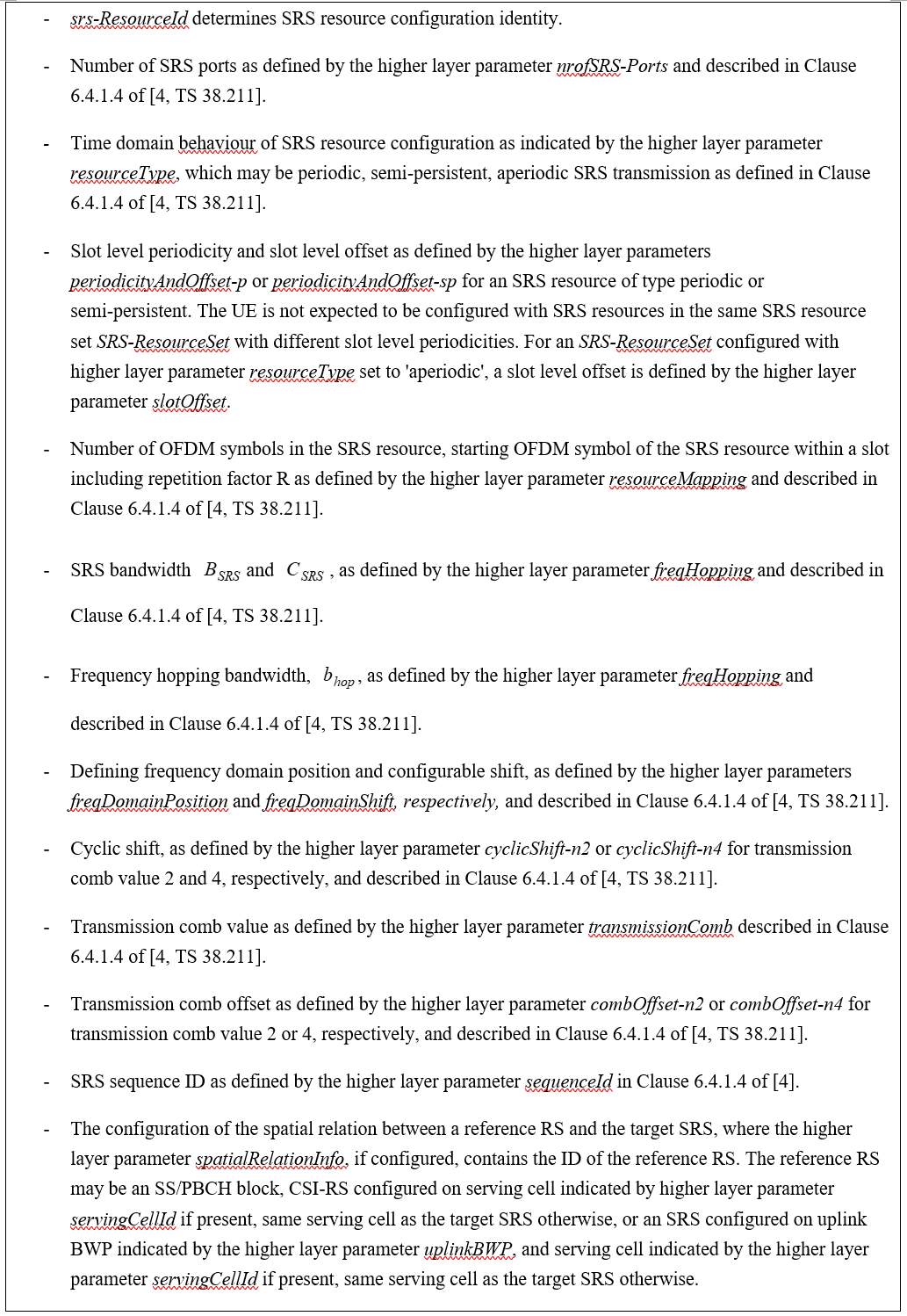UE 由高层参数 SRS-Resource 中的字段 resourceMapping 来配置 SRS 资源，该 SRS 资源位于时隙内最后 6 个符号的区域，并占用 $$N_S\in\left\{1,2,4\right\}$$ 个连续符号。

• 如果配置给 UE 的高层参数spatialRelationInfo 中包含了参考 “ssb-Index”，则 UE 发送目标 SRS 资源时，将使用与接收参考信号 SSB 相同的空间域传输过滤器。

• 如果配置给 UE 的高层参数spatialRelationInfo 中包含了参考 “csi-RS-Index”，则 UE 发送目标 SRS 资源时，将使用与接收周期性 CSI-RS 或者半持续性 CSI-RS 相同的空间域传输过滤器。

• 如果配置给 UE 的高层参数spatialRelationInfo 中包含了参考 “srs”，则 UE 发送目标 SRS 资源时，将使用与接收周期性 SRS 相同的空间域传输过滤器。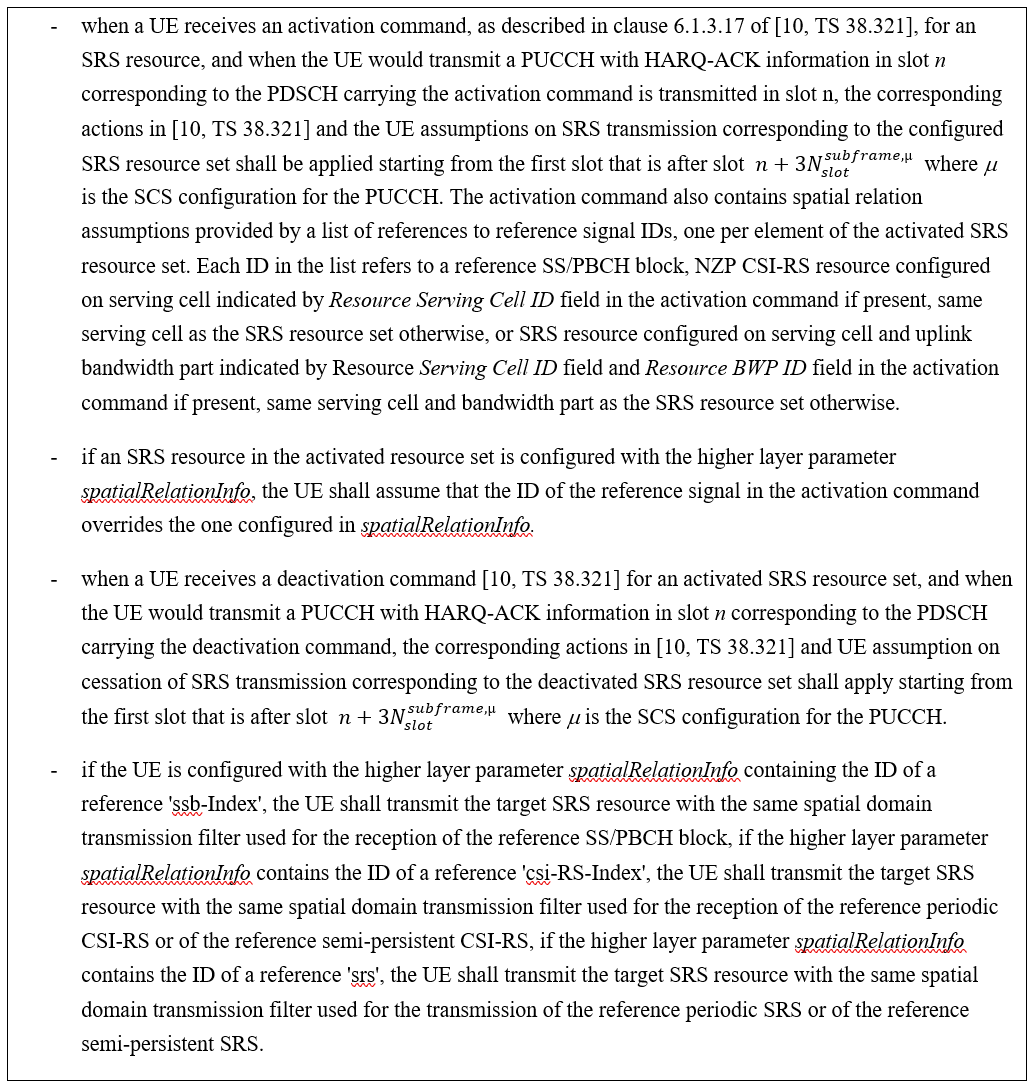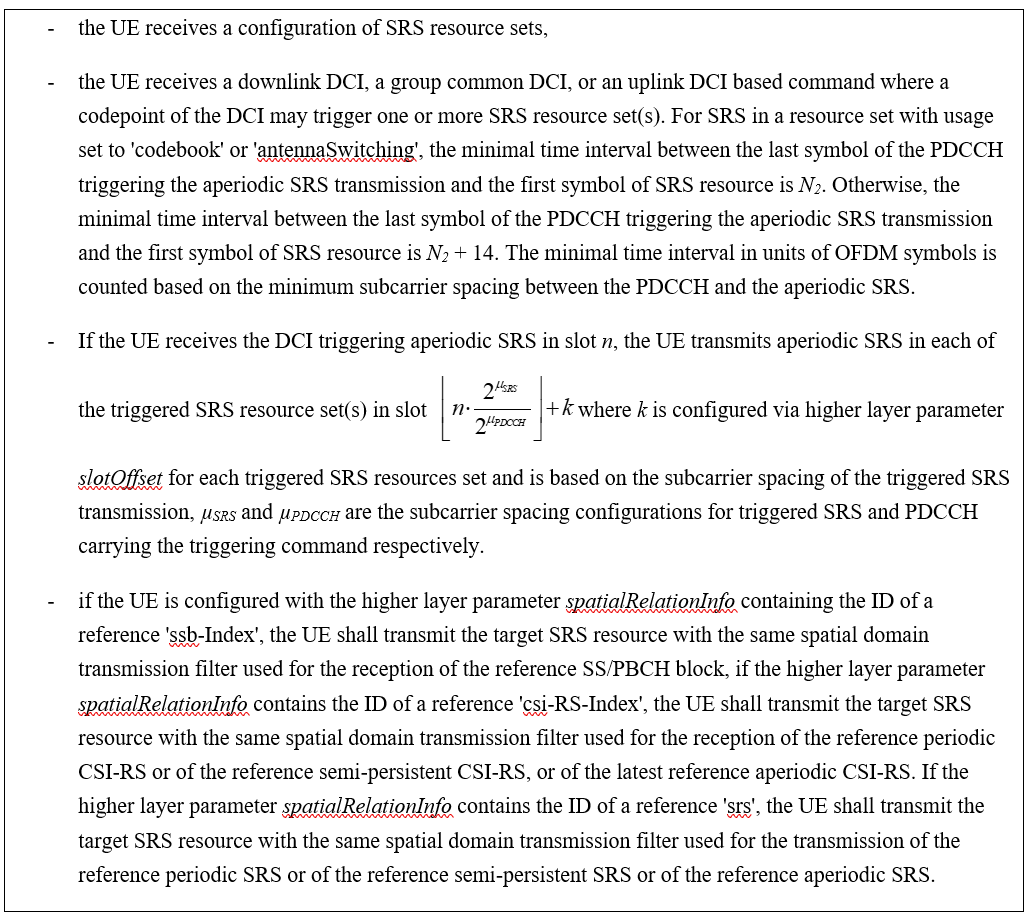DCI format 0_1 和 DCI format 1_1 中的SRS request 字段指示了 Table 7.3.1.1.2-24 中被触发的 SRS 资源集。DCI format 2_3 中 2-bit 的 SRS request 字段，如果 UE 被高层参数 srs-TPC-PDCCH-Group 设置为 “typeB”，则该字段用于指示被触发的 SRS 资源集；如果 UE 被高层参数 srs-TPC-PDCCH-Group 设置为 “typeA”，则该字段用于指示通过高层配置的一组服务小区上进行SRS传输。

# SRS 的冲突处理

PUCCH 和 SRS 在相同载波条件下，

• 当半持续和周期 SRS 与仅携带 CSI 报告的 PUCCH 被配置在相同的符号时，或者当半持续和周期 SRS 与仅携带 L1-RSRP 报告的 PUCCH 被配置在相同的符号时, UE 不应发送 SRS。

• 当半持续性 SRS 或周期性 SRS 或非周期性 SRS 被触发后，与携带 HARQ-ACK 和/或 SR 的 PUCCH 在相同符号发送时，UE 不应发送 SRS。

• 对由于与 PUCCH 重叠而不发送 SRS 的情况，仅丢弃与 PUCCH 重叠的 SRS 符号。

• 非周期性 SRS 被触发后，与携带半持续/周期 CSI 报告或仅携带半持续/周期 L1-RSRP 报告的 PUCCH 在相同符号时，UE 不应发送 PUCCH。

In case of intra-band carrier aggregation or in inter-band CA band combination if simultaneous SRS and PUCCH/PUSCH transmissions are not supported by UE, the UE is not expected to be configured with SRS from a carrier and PUSCH/UL DM-RS/UL PT-RS/PUCCH formats from a different carrier in the same symbol. In case of intra-band carrier aggregation or in inter-band CA band combination if simultaneous SRS and PRACH transmissions are not supported by UE, the UE shall not transmit simultaneously SRS resource(s) from a carrier and PRACH from a different carrier.

## UE SRS 跳频过程

UE可以被配置 Ns = 2 or 4 个相邻符号的非周期 SRS 资源并在 BWP 内进行时隙内跳频，其中当跳频配置为 $$R=1$$ 时，在 $$N_s$$ 个符号上以相等大小的 sub-band 来探测全跳频带宽。UE可以被配置 $$N_s=4$$ 个相邻符号非周期 SRS 资源并在 BWP 内进行时隙内跳频，其中当跳频配置为R = 2时，在两对 R 个相邻的 OFDM 符号之间以相等大小的 sub-band来探测全跳频带宽。SRS 资源的每个天线端口被映射到每对 R 个相邻的 OFDM 符号中相同的子载波集合。

UE可以被配置 $$N_s=1$$ 个符号的周期或半持续 SRS 资源并在 BWP 内进行时隙间跳频，其中 SRS 资源在每个时隙中占据相同的符号位置。UE可以被配置 Ns = 2 or 4 个符号的周期或半持续 SRS 资源并在 BWP 内进行时隙内和时隙间跳频，其中N 个符号的 SRS 资源在每个时隙中占据相同的符号位置。对于 $$N_s=4$$，当跳频配置为 R = 2 时，SRS 资源的每个天线端口通过每个时隙中的资源的两对 R 个相邻的 OFDM 符号映射到不同子载波集合以支持时隙内和时隙间跳频。对于 $$N_s=R$$，当跳频被配置，SRS 资源的每个天线端口映射到每个时隙中的资源的 R 个相邻 OFDM 符号中的相同一组子载波集合以支持时隙间跳频。

## 用于下行 CSI 获取的 UE 探测过程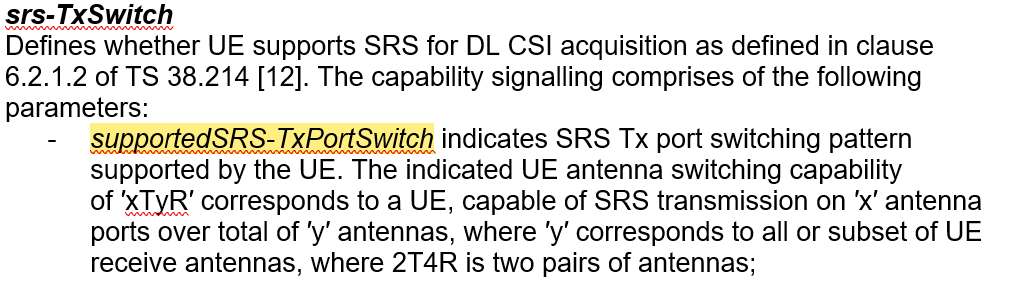• 对于 1T2R，最多配置两个 SRS 资源集。一个 SRS 资源集中包含两个配置在不同 OFDM 符号的 SRS 资源，每个 SRS 资源有 1 个端口。同一个 SRS 资源集中的不同 SRS 资源使用不同的 UE 天线端口（物理天线）分别发送。当配置两个 SRS 资源集时，这两个 SRS 资源集须通过高层参数 resourceType配置为不同的资源类型。

• 对于 2T4R，最多配置带个 SRS 资源集。一个 SRS 资源集中包含两个配置在不同 OFDM 符号的 SRS 资源，每个 SRS 资源有 2 个端口，其中的一个 SRS 资源由两个 UE 天线端口（物理天线）发送，另一个 SRS 资源则从另外两个 UE 天线端口（物理天线）发送。当配置两个 SRS 资源集时，这两个 SRS 资源集须通过高层参数 resourceType配置为不同的资源类型。

• 对于 1T4R，可以配置零个或一个周期或半持续 SRS 资源集。当配置一个周期或半持续 SRS 资源集时，该资源集内包含 4 个 SRS 资源，每个 SRS 资源包含 1 个端口，不同的 SRS 资源配置在不同的 OFDM 符号上，且使用不同的 UE 天线端口（物理天线）发送。

• 对于 1T4R，可以配置零个或两个非周期 SRS 资源集。当配置两个非周期 SRS 资源集时，由于天线切换需要时间，4 个 SRS 资源不能在一个时隙内发完，因此两个非周期 SRS 资源集要在两个时隙内发送。两个 SRS 资源集共包含 4 个 SRS 资源，每个资源集各包含两个 SRS 资源或者一个 SRS 资源集包含 1 个SRS 资源，另外一个 SRS 资源集包含 3 个 SRS 资源，每个 SRS 资源包含一个端口，不同的 SRS 资源通过不同的 UE 物理天线发送。当配置两个非周期 SRS 资源集时，两个 SRS 资源集需配置相同的功率控制参数，包括 SRS-ResourceSet 中的字段 alpha, p0, pathlossReferenceRS 和 srs-PowerControlAdjustmentStates。UE期望高层参数aperiodicSRS-ResourceTrigger的值或每个SRS-ResourceSet中AperiodicSRS-ResourceTriggerList中的条目的值相同，并且每个SRS-ResourceSet中的高层参数slotOffset的值不同 。

• 对于 1T=1R 或 2T=2R 或 4T=4R，最多配置两个 SRS 资源集。每个 SRS 资源集包含 1 个 SRS 资源，每个 SRS 资源的端口数可以为 1，2或4。

Y 的值由 Table 6.2.1.2-1 给出。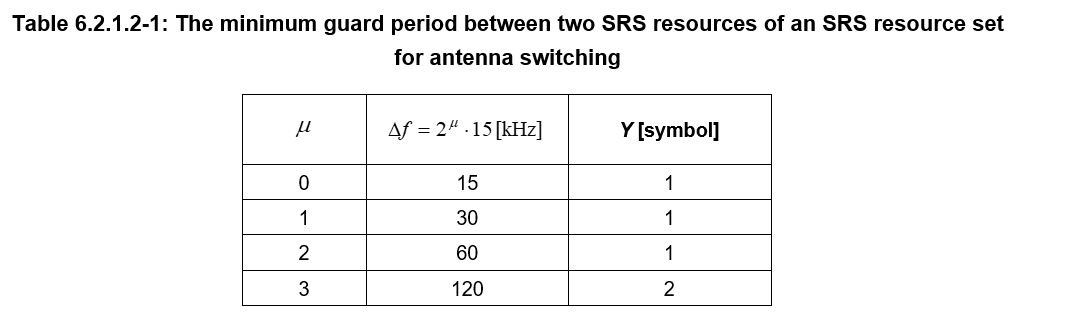# 低峰均比序列

$r_{u,v}^{\left(\alpha,\delta\right)}\left(n\right)=e^{j\alpha n}{\bar{r}}_{u,\nu}\left(n\right), 0≤n<M_{ZC}$

（1）SRS 带宽 4 RB，传输梳齿数目 $$K_{\mathrm{TC}}=2$$, 则 $$\delta={log}_2\left(2\right)=1$$$$\frac{1}{2}\le\frac{4}{2}\le5$$。此时序列长度为 $$M_{\mathrm{ZC}}=\frac{mN_{\mathrm{sc}}^{\mathrm{RB}}}{2^\delta}=4\ast12/2=24$$

（2）SRS 带宽 4 RB，传输梳齿数目 $$K_{\mathrm{TC}}=4$$, 则 $$\delta={log}_2\left(4\right)=2$$$$\frac{1}{2}\le\frac{4}{4}\le5$$。此时序列长度为 $$M_{\mathrm{ZC}}=\frac{mN_{\mathrm{sc}}^{\mathrm{RB}}}{2^\delta}=4\ast12/4=12$$

（3）SRS 带宽 8 RB，传输梳齿数目 $$K_{\mathrm{TC}}=4$$, 则 $$\delta={log}_2\left(4\right)=2$$$$\frac{1}{2}\le\frac{8}{4}\le5$$。此时序列长度为 $$M_{\mathrm{ZC}}=\frac{mN_{\mathrm{sc}}^{\mathrm{RB}}}{2^\delta}=8\ast12/4=24$$

## 长度大于等于 36 的基序列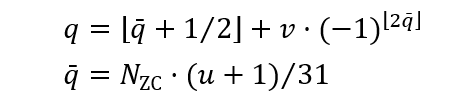ZC 序列长度 $$N_{\mathrm{ZC}}$$ 是满足 $$N_{\mathrm{ZC}}<M_{\mathrm{ZC}}$$ 的最大质数。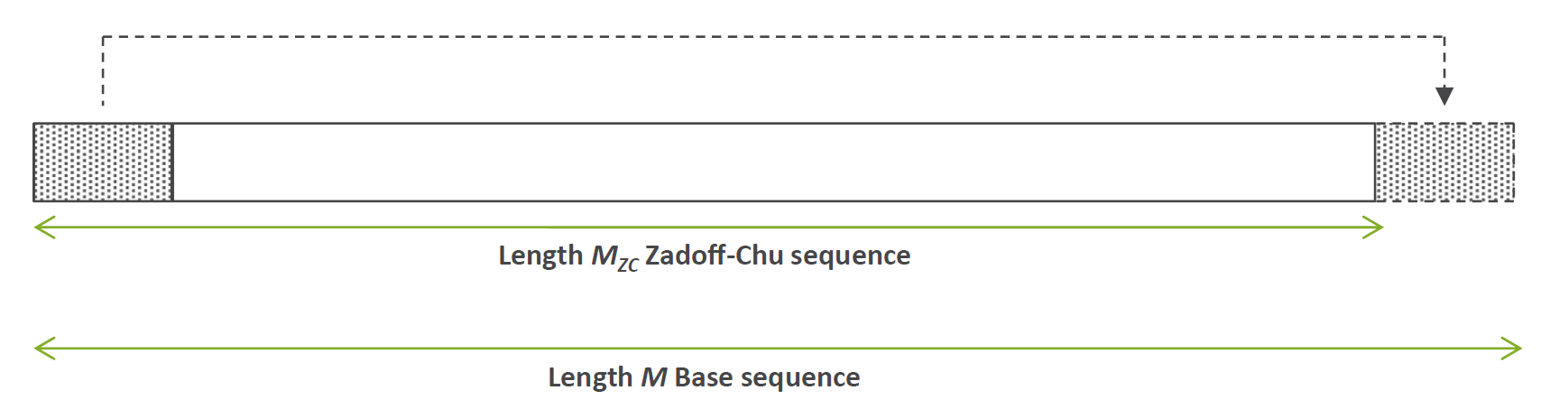ZC 序列的长度通常小于基序列的长度，剩余的那部分基序列由 ZC 序列循环扩展得到，首尾相接。例如基序列长度 $$M_{\mathrm{ZC}}=36$$，则 ZC 序列长度为 $$N_{\mathrm{ZC}}=31$$。通过对基序列进行循环移位可得到更多的 ZC 序列，当 $$N_{\mathrm{ZC}}=31$$ 时，可用的 ZC 序列有 30 个。可用的 ZC 序列数目为 $$N_{\mathrm{ZC}}-1$$

## 长度小于 36 的基序列

${\bar{r}}_{u,\nu}\left(n\right)=\begin{matrix}e^\frac{j\phi\left(n\right)\pi}{4},&0\le n\le M_{\mathrm{ZC}}-1\\\end{matrix}$

${\bar{r}}_{u,\nu}\left(n\right)=\begin{matrix}e^{-j\frac{\pi(u+1)(n+1)(n+2)}{31}},&0\le n\le M_{\mathrm{ZC}}-1\\\end{matrix}$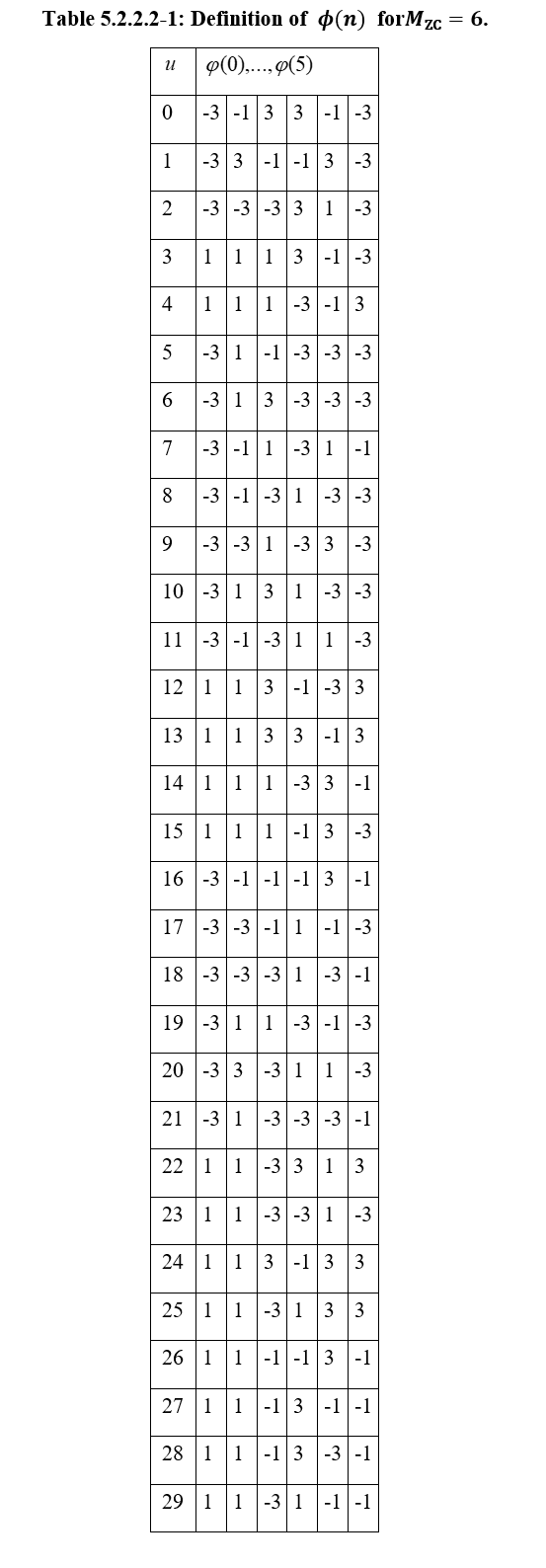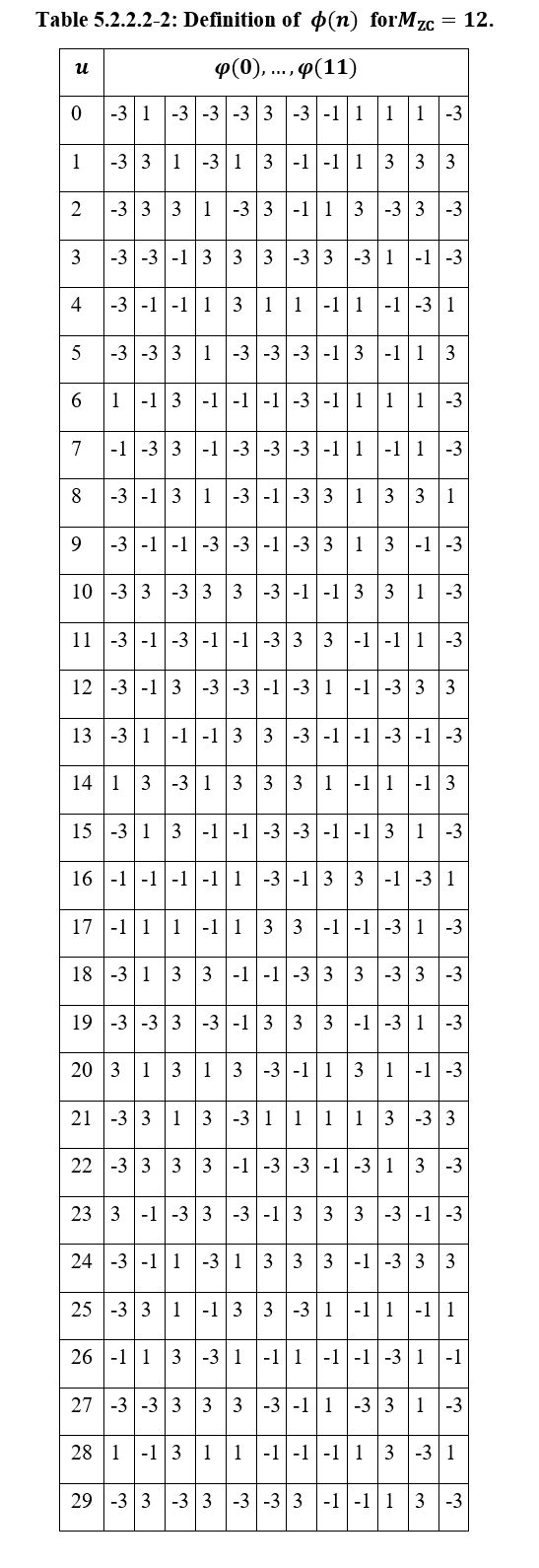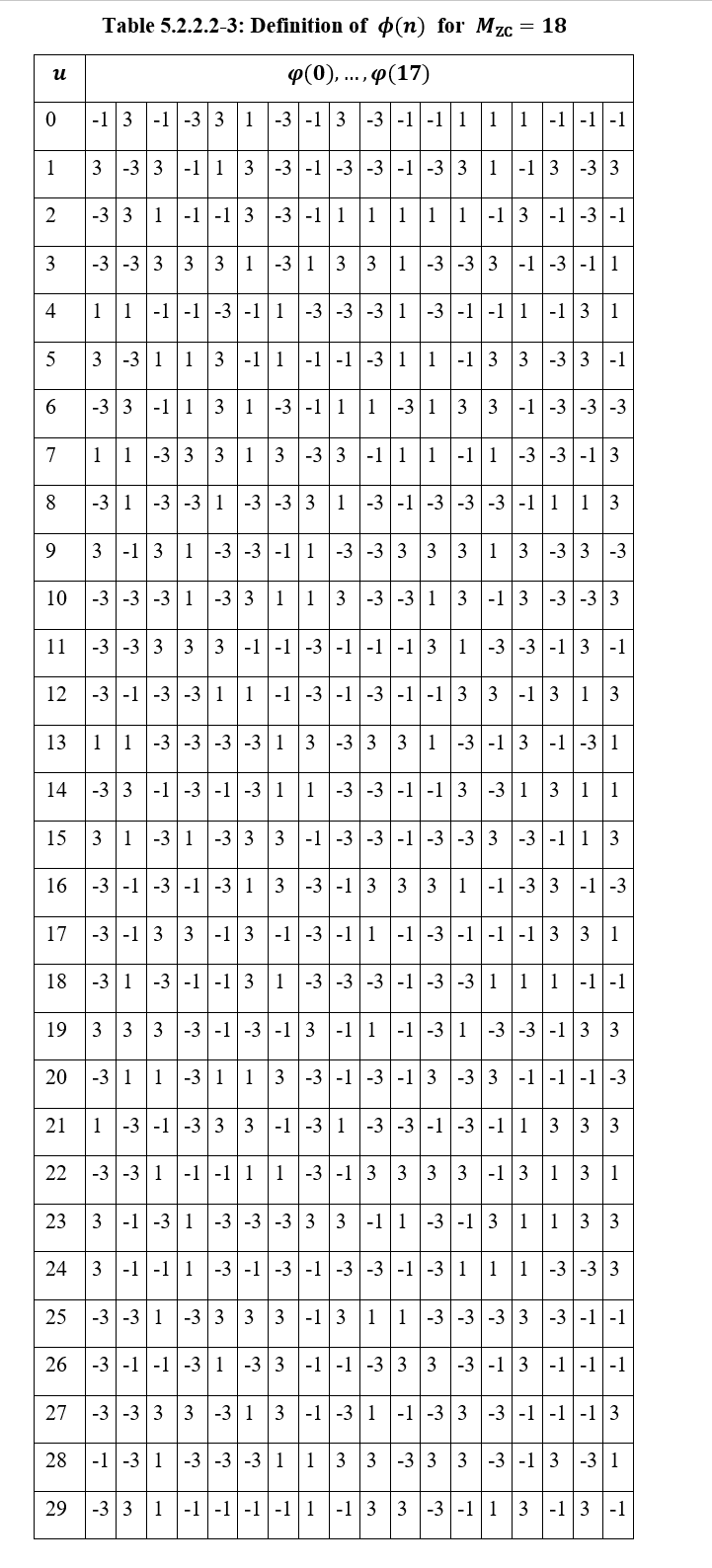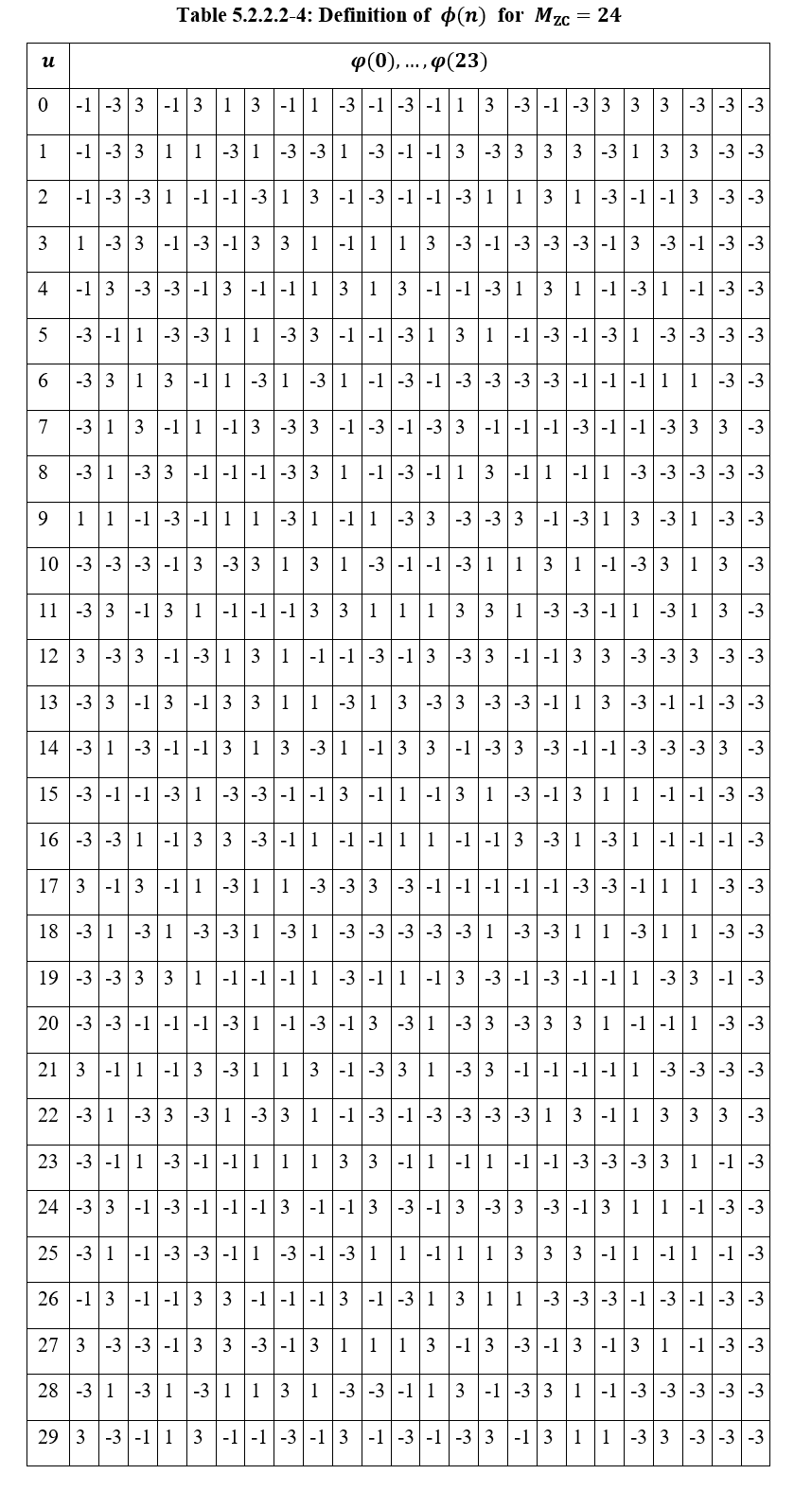# 为什么是 ZC 序列

1962 年 Zadoff 和 Frank 提出了一种循环自相关为零的多相编码（polyphase codes），但编码的长度受限于 p^2。十年后（1972），Chu 基于 Zadoff 和 Frank 的研究成果，提出了一种新的序列构造方法，依然具有完美的零相关性，但序列长度没有限制。这种新的序列就是 Zadoff-Chu 序列。又数十年后，它应用在4G 和 5G 的参考信号中。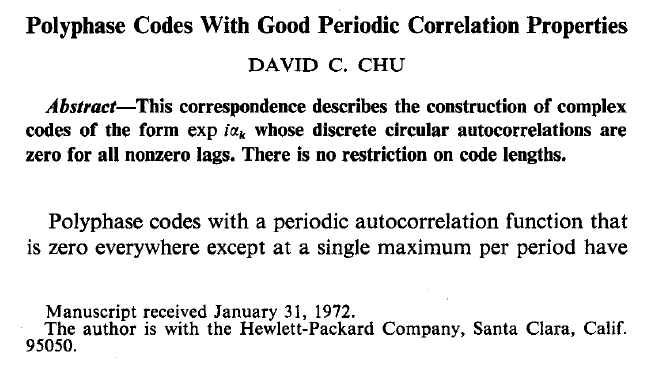David C. Chu 在 1972 年从数学上证明了 Zadoff-Chu 序列具有完美的循环自相关特性。

ZC 序列根据序列长度 N 为偶或奇定义如下：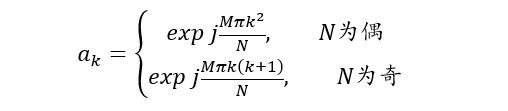$x_0=\sum_{k=0}^{N-1}{a_ka_k^\ast}$

$x_m=\sum_{k=0}^{N-m-1}{a_ka_{k+m}^\ast}+\sum_{k=N-m}^{N-1}{a_ka_{k+m-N}^\ast},\ \ j=1,2,...,N-1$

Output: